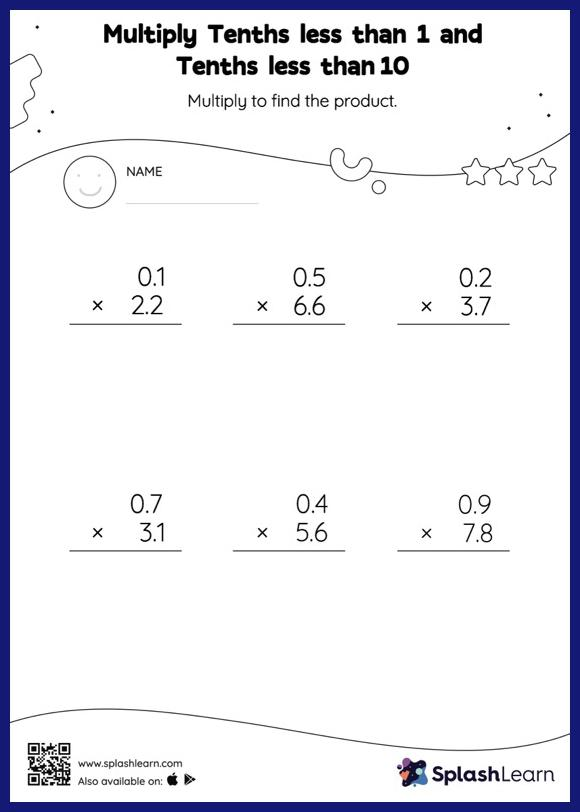# Multiply Tenths less than 1 and Tenths less than 10: Vertical Multiplication Worksheet

Home > Multiply Tenths less than 1 and Tenths less than 10: Vertical MultiplicationDoes your child know how to multiply tenths less than 1 and tenths less than 10? Invite them to practice this concept here. As the worksheet uses the column method, it is helpful in getting students toward higher accuracy, especially with bigger numbers and in scenarios where regrouping is required.